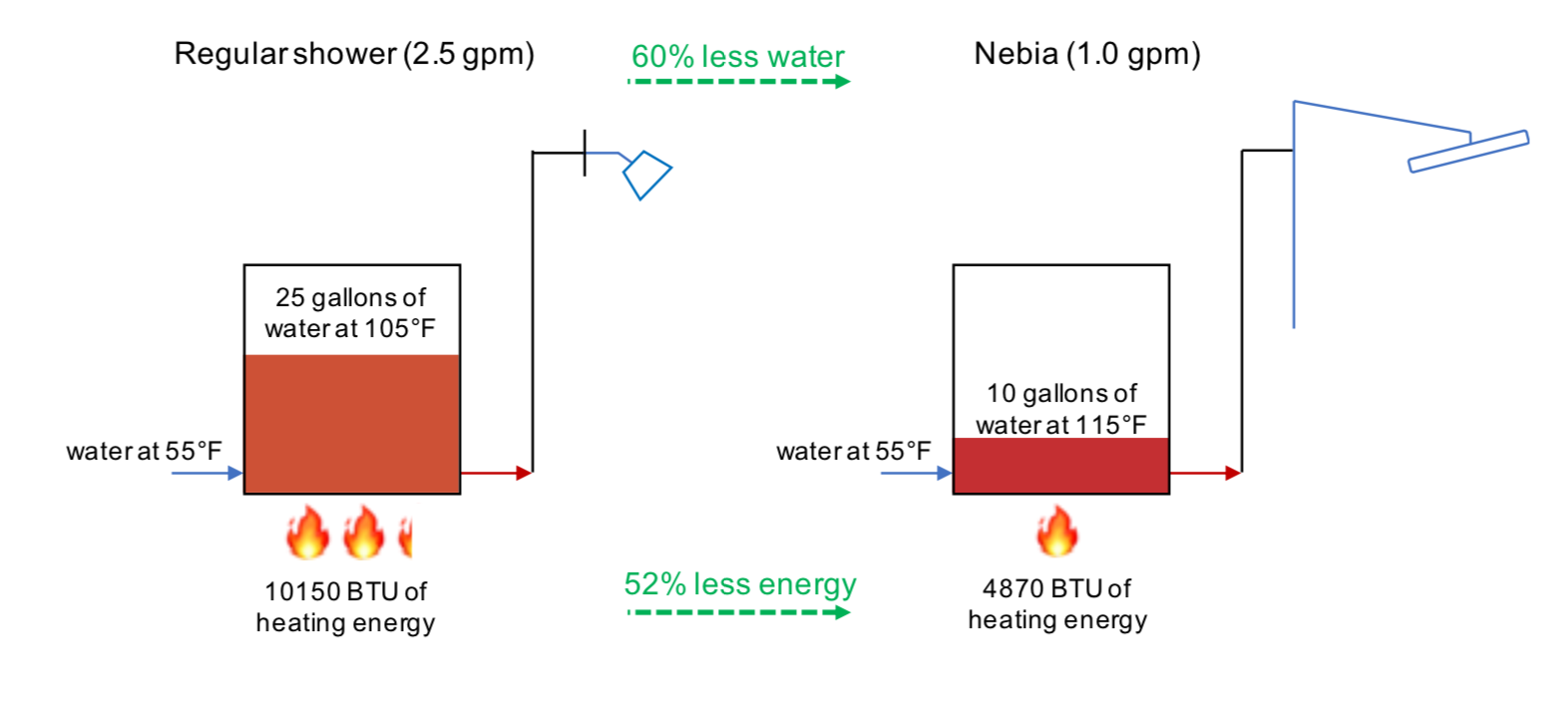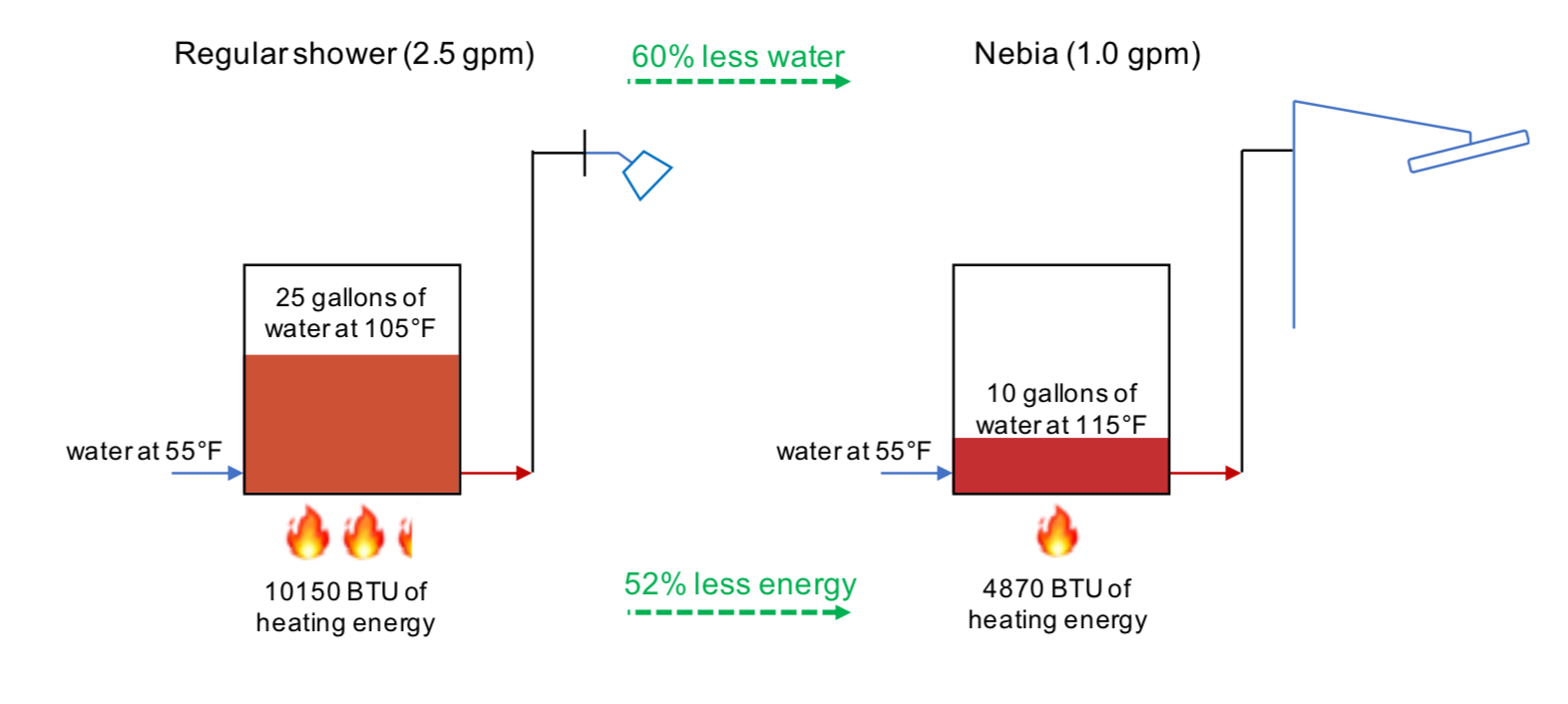Blog > How to Calculate Energy Savings With Nebia

# How to Calculate Energy Savings With NebiaThe following exercise and the accompanying picture are intended to demonstrate how the Nebia Spa Shower 2.0 saves heating energy, despite operating at a higher temperature than a regular shower.

Consider a regular shower that uses 2.5 gallons per minute. Let’s assume Bob takes a 10 minute shower, using 25 gallons of water, at a typical water temperature of 105°F. Let’s also assume that Bob’s municipal water is available at 55°F, which is the US average. The heating energy required from Bob’s hot water heater is therefore the energy required to raise the temperature of 25 gallons of water from 55°F to 105°F, or 50°F.

The energy required to increase the temperature of water, E, is equal to

m c △ T

where is the mass of water, is the heat capacity of water (a constant), and △is the temperature change, in degrees. Ignoring the constant c, the water required to heat 25 gallons of water by 50°F, in arbitrary units, is 25 * 50 = 1250 units.

Now consider Bob takes a Nebia shower with a flow rate of 1.0 gallons per minute. Bob showers for 10 minute, using 10 gallons of water (instead of his normal 25 gallons). Because Nebia works best with higher water temperature than a regular shower, Bob prefers to run his Nebia with a water temperature of 115°F, instead of 105°F. In this case, Bob’s hot water heater must raise the temperature of 10 gallons of water from 55°F to 115°F, or 60°F. The amount of heating energy, in the same imaginary units as before, is 10 * 60 = 600 units of energy.

In this example, Bob’s water savings are (2.5–1.0)/2.5 = 60%, and his heat savings are (1250–600)/1250 = 52%.

The key here is that the energy required to heat water is proportional to both the amount of water and the magnitude of the temperature increase. While the required temperature increase is greater for Nebia than for a regular shower, the amount of water being heated is much less than the amount of heating energy required, with the end result of significant heat savings.